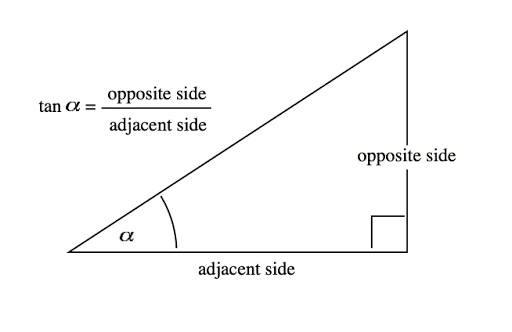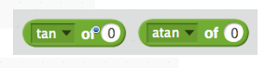## Monday, February 22, 2021

### Make a Block II - The Recursive Koch Curve

How to use Make a Block to program the famous Koch curve is the topic of this post. Below is a graphic that shows the stages (levels) of the curve's development. Instead of writing a script for each level, Make a Block and the programming technique known as recursion allows the program to be created using a single[ define recursive call side length level]script.

Here's the working script. With side length = 297 and level as the only other input, this script will draw figures 1 through 5 as shown above.
Teachers and/or students might like a free PDF file that steps through the development of the script shown above. The technique used in this Scratch program is applicable to many other similarity fractals like the Koch curve. It might interest you to know that mathematics still does not have an agreed upon definition for curve
If any of the levels shown in the diagram above are drawn on the sides of an equilateral triangle the result is know as a Koch Snowflake. It can be shown that as the perimeter of the curve approaches infinity the area approaches 8/5 the area of the original equilateral triangle.

Teachers and/or students might also be interested in the free PDF document that describes the formula for the perimeter of the curve as a function of level.
Either or both of the documents mentioned above can be obtained by sending an email request to grandadscience@gmail.com. I send my free PDF documents to teachers and students all over the world and I have never had a single complaint.

## Thursday, February 11, 2021

### How to Use Make a Block in Scratch

Scratch 2.0 introduced the Make a Block option that made recursion easy to implement in Scratch.
The mechanics of using Make a Block need explaining as the process for making a block and how variables are handled in Make a Block are different than the mechanics for creating a variable under the Orange variable menu.
When you click on the Make a Block option the following menu appears.

Note that the define top block is red with the name square and the variable side length also in red. The arrow indicates the variable used in the move block is taken from the define block itself and not the orange variable menu. The orange and blue blocks were taken from their regular menus.
This Make a block was used to create ten squares, each a different color and side length.

The purpose of this project is to illustrate the mechanics of using Make a Block. A later post will focus on the programming power of Make a Block.
Teachers and students might be interested in a free PDF tutorial that describes this project in more detail. The tutorial can be obtained by emailing a request to www.grandadscience@gmail.com.
You can also view and download another Make a Block project by clicking on the following link.

## Monday, January 25, 2021

### The Sine of 35º is a Constant

It is well known that the ratio of the circumference to the diameter of any circle equals Pi (π).
The circle could be as small as that of an atom or as large as a circle in space with a diameter of one light year. In all such cases the ratio of the circumference to the diameter is constant. The ratio of the circumference to the diameter in the red circle is the same for the yellow and black circles.

Good math teachers will have students measure the circumference of several circular objects (like circular lids) as accurately as possible to establish the belief that the ratio of the circumference to the diameter of any circle is Pi.
I remember being taught the sine, cosine, and tangent trigonometry functions from a single textbook diagram of a right triangle. No effort was made to show that the ratios were constant for any given angle no matter the size of the right triangle containing the given angle.

This project has the mouse draw a right triangle with angles equal to 35º, 90º, and 55º. The mouse first draws the hypotenuse of the right triangle a random number of steps. The mouse turns to the right and draws the perpendicular to the base of the triangle. The mouse then turns right through 90º and draws the side adjacent to the 35º angle. The program then computes and displays the sine(35º) using the known lengths of the hypotenuse and opposite side. The program repeats’ this process 9 more times, producing a series of right triangles that demonstrate the sine(35º) is a constant 0.57735… for all of the ten right triangles.

This is a screen shot of the output of the project.

Teachers and students might be interested in a free PDF tutorial on the project that can be obtained by emailing www.grandadscience@gmail.com.

## Thursday, January 14, 2021

### Scalene Triangle Mouse Using the Law of Cosines

In the December 21, 2020 post (Coding Math Concepts as Tests of Student Comprehension) I presented the problem of coding a scalene triangle as a challenge considerably tougher than just learning the definition of a scalene triangle and examining a few triangles.
I felt there was a crudeness to the method I used and even published an Addendum December 23, 2020 (Addendum to Scalene Triangle Mouse I) that 'fixed' the heading problem presented by the go to that drew the third leg. In this post, I offer another method for drawing a scalene triangle that uses the law of cosines taught in s first trigonometry course.

The method is straight forward and essentially requires coding the angle formulas shown in the following diagram since the program asks the user to set the lengths of the three sides to different values (the definition).

Teachers and students might be interested in the free PDF file that describes the Scratch program in more detail. If interested, send an email requesting Scalene Triangle Mouse II and I will end you a copy that holds no copyright. I will also send upon request the Word file that can be changed if you don't have Adobe.

## Monday, January 11, 2021

### Using Lists in Scratch - Singe Die Frequencies

Scratch is a descendent of the Logo programming language that was developed by Dr. Seymour Papert at the the Artificial Lab at MIT. Logo had elements of the LISP programming language popular at the Lab at that time. LISP is an acronym for LIStProcessing and used data structures called lists.
To illustrate the use of lists I've written a PDF document that uses lists to store the frequency of the results of throwing a single die a number of times. Assuming the die is a fair die, the frequency of each of the numbered faces, 1, 2, 3, 4, 5 and 6 should be equal for say 6 throws, 36 throws, and 216 throws. Here is the Scratch script.

Below is a screenshot of the display produced by the above script for six rolls of a single die. Note that the program does not produce the theoretical values of the frequency of 1 for each of the six possibilities. Bit neither does six rolls of a real die.   If you are a teacher or a students and would like a free copy of the PDF file that details the building of the script just email your request to grandadscience@gmail.com.
A more advanced project that uses lists to record the frequency of the sum of a pair of dice for a repeated number of throws will be the topic of a future post.

## Wednesday, December 23, 2020

### Addendum to Scalene Triangle Mouse I

At the conclusion of the Coding the Scalene Triangle Mouse I document, I described what I considered to be a flaw in the code; when completing the third leg of the triangle the mouse did not actually point to the starting point of the triangle but moved in a skewed fashion.
Scratch does contain a [point towards (mouse-pointer or another sprite)] block.

I suppose one could make the starting point of the triangle a small circular sprite and then simply ‘point to it’ to draw the third leg of the triangle. I call this approach a work-around as it solves the problem for the present but when time allows a more elegant solution will be found. So I thought about how to create code that will point the mouse from one point to any other specified point. In other words, I wanted to code a generalized solution. It turns out that the basic trigonometry operator, tangent (angle) abbreviated tan(angle) helps to solve the problem.In Scratch, the tan operator (see below) returns the numerical ratio of the opposite side over adjacent side as shown in the diagram above.
The arctan operator takes the numerical ratio and returns the angle.
For example, for a 38º angle, tan 38º = 0.7812 and the arctan of 0.7812 = 38ºIn the diagram below the xpos and ypos reporters in Scratch return the values for x2 and y2. The values for x1 and y1 are set in the code. These values are used to compute the tangent of the angle and then the arctangent operator computes the value in degrees of the angle. The mouse can then be turned to the left through the angle (90º – angle)  to be pointed directly towards the point (x1, y1).

I have written a detailed tutorial describing the coding of the above addendum to the Scalene Mouse Triangle project. A free PDF file of this document that might be useful to both teachers and students can be obtained by emailing me at grandadscience@gmail.com.

## Monday, December 21, 2020

### Pen Commands in Scratch 3

Go to http://scratch.mit.edu/ and click on Create in the upper left corner. The Editor page, shown below, will open. This version is Scratch 3. Earlier versions of Scratch had a Pen tab listed with the other colored categories of blocks. Scratch 3 has moved the Pen commands.
To find them, note the small blue block in the lower left of the Scratch home screen. The blue icon indicates additional blocks. Click on the blue icon and a page of additonal commands are revealed.

Click on the Pen icon in the lower left corner of the above menu to reveal a page of options. Choose the option Pen.
If you use Pen commands in a project, every time you open the project the Pen icon will appear under the My Blocks menu icon.

A copy of this post in PDF file format suitable for teacher or student use can be obtained, free of charge, by sending an email request to grandadscience@gmail.com.